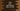# Java program to print odd numbers from 1 to 100## Java program to print odd numbers from 1 to 100:

This post will show you how to print odd numbers in Java from 1 to 100 or in a given range. With this program, you will learn how to read user inputs in Java, how to use a loop and how to print output on the console.

### How to find if a number is odd:

A number is odd if it is not divisible by 2, i.e. the remainder is not 0 if we divide it by 2. For example, 3, 5, 7 are odd numbers.

We can use the modulo operator, % to find out the remainder. x%y will return the remainder of x divided by y or x/y. We can compare this remainder with 0 to find out if a number is odd or not.

### Example 1: Java program to print odd numbers from 1 to 100 using a for loop:

In this example, we will write one program that will print all odd numbers from 1 to 100 by using a for loop.

``````public class Main {
public static void main(String[] args) {
System.out.println("Odd numbers between 1 to 100 are: ");

for (int i = 1; i <= 100; i++) {
if (i % 2 != 0) {
System.out.print(i + " ");
}
}
}
}``````
• This program uses a for loop to find out all odd numbers between 1 to 100.
• The loop runs from 1 to 100.
• On each iteration, it checks if the current value of i is odd or not. If yes, it prints its value.

If you run this program, it will print:

``````Odd numbers between 1 to 100 are:
1 3 5 7 9 11 13 15 17 19 21 23 25 27 29 31 33 35 37 39 41 43 45 47 49 51 53 55 57 59 61 63 65 67 69 71 73 75 77 79 81 83 85 87 89 91 93 95 97 99 ``````

### Example 2: Java program to print odd numbers from 1 to 100 using a while loop:

Let’s write down the above program by using a while loop. It works similar to the for loop. But, it checks for the condition first before it enters to the loop body.

``````public class Main {
public static void main(String[] args) {
System.out.println("Odd numbers between 1 to 100 are: ");

int i = 1;
while (i <= 100) {
if (i % 2 != 0) {
System.out.print(i + " ");
}
i++;
}
}
}``````

It will print the same output.

``````Odd numbers between 1 to 100 are:
1 3 5 7 9 11 13 15 17 19 21 23 25 27 29 31 33 35 37 39 41 43 45 47 49 51 53 55 57 59 61 63 65 67 69 71 73 75 77 79 81 83 85 87 89 91 93 95 97 99 ``````
• The integer i is initialized as 1 before the loop starts.
• On each iteration, it checks if it is odd or not. If yes, it prints its value.
• At the end of each iteration, it increments the value of i by 1.
• The loop will run until the value of i is less than or equal to 100.

### Example 3: Java program to print odd numbers in any range:

Let’s take the start and end values of the range as inputs from the user. This program will print the odd numbers in any given range:

``````import java.util.Scanner;

public class Main {
public static void main(String[] args) {
int start, end;
Scanner scanner = new Scanner(System.in);

System.out.println("Enter the start value of the loop: ");
start = scanner.nextInt();

System.out.println("Enter the end value of the loop: ");
end = scanner.nextInt();

System.out.println("Odd numbers between " + start + " and " + end + " are:");

for (int i = start; i <= end; i++) {
if (i % 2 != 0) {
System.out.print(i + " ");
}
}
}
}``````
• The Scanner object is used to read user inputs. It asks the user to enter the start and end values of the range, reads the inputs and assigns these to the start and end integer variables.
• The for loop runs from start to end and on each iteration it checks for odd similar to the previous example.

If you run this program, it will print output as below:

``````Enter the start value of the loop:
1
Enter the end value of the loop:
10
Odd numbers between 1 and 10 are:
1 3 5 7 9 ``````